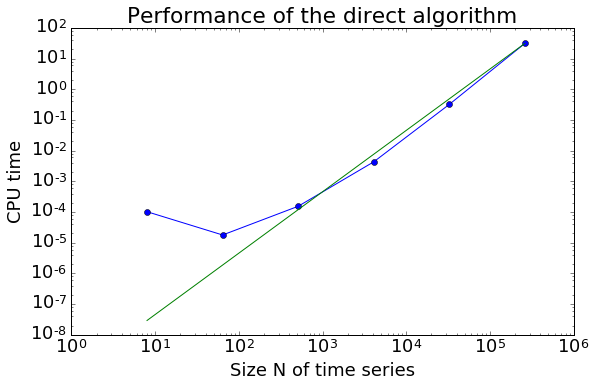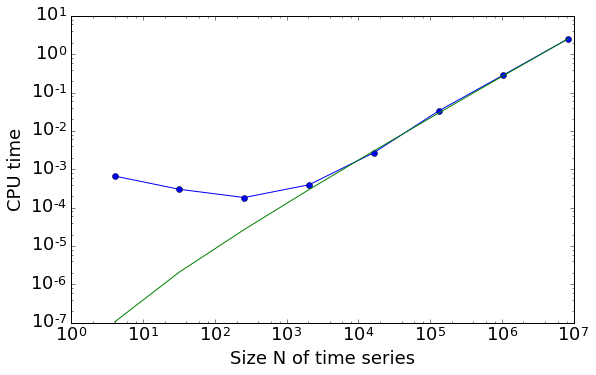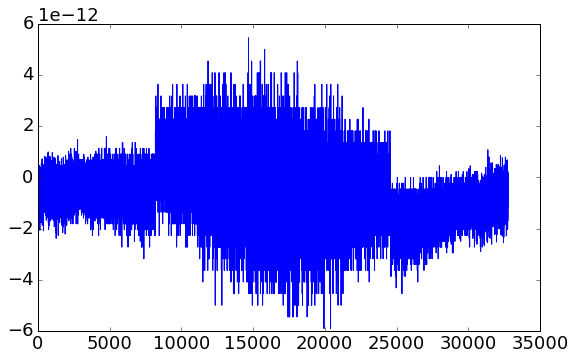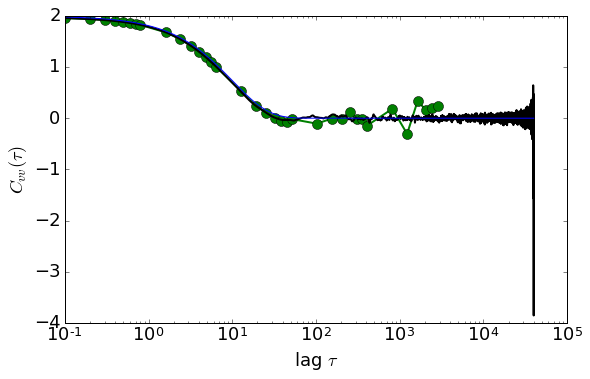## Correlators for molecular and stochastic dynamics

Time correlations represent one of the most important data that one can obtain from doing molecular and stochastic dynamics. The two common methods to obtain them is via either post-processing or on-line analysis. Here I review several algorithms to compute correlation from numerical data: naive, Fourier transform and blocking scheme with illustrations from Langevin dynamics, using Python.

### Introduction¶

I conduct molecular and stochastic simulations of colloidal particles. One important quantity to extract from these simulations is the autocorrelation of the velocity. The reasoning also applies to other types of correlation functions, I am just focusing on this one to provide context.

There are several procedures to compute the correlation functions from the numerical data but I did not find a synthetic review about it, so I am making my own. The examples will use the Langevin equation and the tools from the SciPy stack when appropriate.

We trace the apparition of the methods using textbooks on molecular simulation when available or other references (articles, software documentation) when appropriate. If I missed a foundational reference, let me know in the comments or by email.

In 1987, Allen and Tildesley mention in their book Computer Simulation of Liquids [Allen1987] mention the direct algorithm for the autocorrelation $$C_{AA}(\tau = j\Delta t) = \frac{1}{N-j} \sum_i A_i A_{i+j}$$ where I use $A_i$ to denote the observable $A$ at time $i\Delta t$ ($\Delta t$ is the sampling interval). Typically, $\tau$ is called the lag time or simply the lag. The number of operations is $O(N_\textrm{cor}N)$, where $N$ is the number of items in the time series and $N_\textrm{cor}$ the number of correlations points that are computed. By storing the last $N_\textrm{cor}$ value of $A$, this algorithm is suitable for use during a simulation run. Allen and Tildesley then mention the Fast Fourier Transform (FFT) version of the algorithm that is more efficient, given its scaling in terms of $N\log N$. The FFT algorithm is based on the convolution theorem: performing the convolution (of the time-reversed data) in frequency space is a multiplication and much faster than the direct algorithm. The signal has to be zero-padded to avoid circular correlations due to the finiteness of the data. The requirements of the FFT method in terms of storage and the number of points to obtain for $C_{AA}$ influence what algorithm gives the fastest result.

Frenkel and Smit in their book Understanding Molecular Simulation [Frenkel2002] introduce what they call an "Order-n algorithm to measure correlations". The principle is to store the last $N_\textrm{cor}$ values for $A$ with a sampling interval $\Delta t$ and also the last $N_\textrm{cor}$ values for $A$ with a sampling interval $l \Delta t$ where $l$ is the block size, and recursively store the data with an interval $l$, $l^2$, $l^3$, etc. In their algorithm, the data is also averaged over the corresponding time interval and the observable is thus coarse-grained during the procedure.

A variation on this blocking scheme is used by Colberg and Höfling [Colberg2011], where no averaging is performed. Ramírez, Sukumaran, Vorselaars and Likhtman [Ramirez2010] propose a more flexible blocking scheme in which the block length and the duration of averaging can be set independently. They provide an estimate of the systematic and statistical errors induced by the choice of these parameters. This is the multiple tau correlator.

The "multiple-tau" correlator has since then been implemented in two popular Molecular Dynamics package:

The direct and FFT algorithms are available in NumPy and SciPy respectively.

In the field of molecular simulation, the FFT method was neglected for a long time but was put forward by the authors of the nMOLDYN software suite to analyze Molecular Dynamics simulation [Kneller1995].

### The direct algorithm and the implementation in NumPy¶

The discrete-time correlation $c_j$ from a numerical time series $s_i$ is defined as

$$c_j = \sum_i s_{i} s_{i+j}$$

where $j$ is the lag in discrete time steps, $s_i$ is the time series and the sum runs over all available indices. The values in $s$ represent a sampling with a time interval $\Delta t$ and we use interchangeably $s_i$ and $s(i\Delta t)$.

Note that this definition omits the normalization. What one is interested in is the normalized correlation function

$$c'_j = \frac{1}{N-j} \sum_i s_{i} s_{i+j}$$

NumPy provides a function numpy.correlate that computes explicitly the correlation of a scalar time series. For small sets of data it is sufficiently fast as it is actually calling a compiled routine. The time it takes grows quadratically as a function of the input size, which makes it unsuitable for many practical applications.

Note that the routine computes the un-normalized correlation function and that forgetting to normalize the result, or normalizing them with $N$ instead of $N-j$ will give incorrect results. numpy.correlate (with argument mode='full' returns an array of length $2N-1$ that contains the negative times as well as the positive times. For an autocorrelation, half of the array can be discarded.

Below, we test the CPU time scaling with respect to the input data size and compare it with the expected $O(N^2)$ scaling, as the method computes all possible $N$ values for the correlation.

In :
n_data = 8*8**np.arange(6)
time_data = []
for n in n_data:
data = np.random.random(n)
t0 = time.time()
cor = np.correlate(data, data, mode='full')
time_data.append(time.time()-t0)
time_data = np.array(time_data)

In :
plt.plot(n_data, time_data, marker='o')
plt.plot(n_data, n_data**2*time_data[-1]/(n_data[-1]**2))
plt.loglog()
plt.title('Performance of the direct algorithm')
plt.xlabel('Size N of time series')
plt.ylabel('CPU time')

Out:
<matplotlib.text.Text at 0x7f1285affa90>### SciPy's Fourier transform implementation¶

The SciPy package provides the routine scipy.signal.fftconvolve for FFT-based convolutions. As for NumPy's correlate routine, it outputs negative and positive time correlations, in the un-normalized form.

SciPy relies on the standard FFTPACK library to perform FFT operations.

Below, we test the CPU time scaling with respect to the input data size and compare it with the expected $O(N\log N)$ scaling. The maximum length for the data is already an order of magnitude larger than for the direct algorithm, the CPU time would be already too much for this.

In :
n_data = []
time_data = []
for i in range(8):
n = 4*8**i
data = np.random.random(n)
t0 = time.time()
cor = scipy.signal.fftconvolve(data, data[::-1], mode='full')
n_data.append(n)
time_data.append(time.time()-t0)
n_data = np.array(n_data)
time_data = np.array(time_data)

In :
plt.plot(n_data, time_data, marker='o')
plt.plot(n_data, n_data*np.log(n_data)*time_data[-1]/(n_data[-1]*np.log(n_data[-1])))
plt.loglog()
plt.xlabel('Size N of time series')
plt.ylabel('CPU time')

Out:
<matplotlib.text.Text at 0x7f12856fc2e8>### Comparison of the direct and the FFT approach¶

It is important to note, as mentioned in [Kneller1995] that the direct and FFT algorithms give the same result, up to rounding errors. This is show below by plotting the substraction of the two signals.

In :
n = 2**14
sample_data = np.random.random(n)

direct_correlation = np.correlate(sample_data, sample_data, mode='full')
fft_correlation = scipy.signal.fftconvolve(sample_data, sample_data[::-1])

plt.plot(direct_correlation-fft_correlation)

Out:
[<matplotlib.lines.Line2D at 0x7f1285828518>]### Blocking scheme for correlations¶

Both schemes mentioned above require a storage of data that scales as $O(N)$. This includes the storage of the simulation data and the storage in RAM of the results (at least during the computation, it can be truncated afterwards if needed).

An alternative strategy is to store blocks of correlations, each successive block representing a coarser version of the data and correlation. The principle behind the blocking schemes is to use a decreasing time resolution to store correlation information for longer times. As the variations in the correlation typically decay with time, it makes sense to store less and less detail.

There are nice diagrams in the references cited above but for the sake of completeness, I will try my own diagram here.

• The black full circle in the signal ("s") is taken at discrete time $i=41$ and fills the $b=0$ block at position $41\mod 6 = 5$.
• The next point is the empty circle, it fills the block $b=0$ in position $42\mod 6=0$. As $42\mod l^b$ (for $l=6$ and $b=0$), the empty circle is also copied to position $1$ in the block $b=1$ at position $42 / l^b\mod l=1$ (for $b=1).In the following, •$l$is the length of the blocks •$B$is the total number of blocks The signal blocks contain samples of the signal, limited in time and at different timescales. The procedure to fill the signal blocks is quite simple: 1. Store the current value of the signal,$s_i$into the 0-th signal block, at the position$i\mod l$. 2. Once every$l$steps (that is when$i\mod l=0$), store the signal also in the next block, at position$i/l$3. Apply this procedure recursively up to block$B-1$, dividing$i$by$l$at every step Example of the application of step 3: store the signal in block$b$when when$i\mod l^b=0$, that is every$l$steps for block 1, every$l^2$steps for block 2, etc. The time of sampling for the blocks is 0, 1, 2, etc for block 0, then$0$,$l$,$2l$, etc for block 1 and so on. The procedure to compute the correlation blocks is to compute the correlation of every newly added data point with the$l-1$other values in the same block and with itself (for lag 0). This computation is carried out at the same time that the signal blocks are filled, else past data would have been discarded. This algorithm can thus be executed online, while the simulation is running, or while reading the data file in a single pass. I do not review the different averaging schemes, as they do not change much to the understanding of the blocking schemes. For this, see [Ramirez2010]. The implementation of a non-averaging blocking scheme (compare to papers) is provided below in plain Python. In : def mylog_corr(data, B=3, l=32): cor = np.zeros((B, l)) val = np.zeros((B, l)) count = np.zeros(B) idx = np.zeros(B) for i, new_data in enumerate(data): for b in range(B): if i % (l**b) !=0: # only enter block if i modulo l^b == 0 break normed_idx = (i // l**b) % l val[b, normed_idx] = new_data # fill value block # correlate block b # wait for current block to have been filled at least once if i > l**(b+1): for j in range(l): cor[b, j] += new_data*val[b, (normed_idx - j) % l] count[b] += 1 return count, cor  ### Example with Langevin dynamics¶ Having an overview of the available correlators, I now present a use case with the Langevin equation. I generate data using a first-order Euler scheme (for brevity, this should be avoided in actual studies) and apply the FFT and the blocking scheme. The theoretical result$C(\tau) = T e^{-\gamma \tau}$is also plotted for reference. In : N = 400000 x, v = 0, 0 dt = 0.01 interval = 10 T = 2 gamma = 0.1 x_data = [] v_data = [] for i in range(N): for j in range(interval): x += v*dt v += np.random.normal(0, np.sqrt(2*gamma*T*dt)) - gamma*v*dt x_data.append(x) v_data.append(v) x_data = np.array(x_data) v_data = np.array(v_data)  In : B = 5 # number of blocks l = 8 # length of the blocks c, cor = mylog_corr(v_data, B=B, l=l)  In : for b in range(B): t = dt*interval*np.arange(l)*l**b plt.plot(t[1:], cor[b,1:]/c[b], color='g', marker='o', markersize=10, lw=2) fft_cor = scipy.signal.fftconvolve(v_data, v_data[::-1])[N-1:] fft_cor /= (N - np.arange(N)) t = dt*interval*np.arange(N) plt.plot(t, fft_cor, 'k-', lw=2) plt.plot(t, T*np.exp(-gamma*t)) plt.xlabel(r'lag$\tau$') plt.ylabel(r'$C_{vv}(\tau)$') plt.xscale('log')### Summary¶ The choice of a correlator will depend on the situation at hand. Allen and Tildesley already mention the tradeoffs between disk and RAM memory requirements and CPU time. The table belows reviews the main practical properties of the correlators. "Online" means that the algorithm can be used during a simulation run. "Typical cost" is the "Big-O" number of operations. "Accuracy of data" is given in terms of the number of sampling points for the correlation value$c_j$. Algorithm Typical cost Storage Online use Accuracy of data Direct$N^2$or$N_\textrm{cor}$N$N_\textrm{cor}$for small$N_\textrm{cor}N-j$points for$c_j$FFT$N\log NO(N)$no$N-j$points for$c_j$Blocking$N\ BN\ B$yes$N/l^b$points in block$b$A distinct advantage of the direct and FFT methods is that they are readily available. If your data is small (up to$N\approx 10^2 - 10^3\$) the direct method is a good choice, then the FFT method will outperform it significantly.

An upcoming version of the SciPy library will even provide the choice of method as a keyword argument to the method scipy.signal.correlate, making it even more accessible.

Now, for the evaluation of correlation functions in Molecular Dynamics simulations, the blocking scheme is the only practical solution for very long simulations for which both short- and long-time behaviours are of interest. To arrive in RMPCDMD!

• [Allen1987] M. P. Allen and D. J. Tildesley, Computer Simulation of Liquids (Clarendon Press, 1987).
• [Frenkel2002] D. Frenkel and B. Smit, Understanding molecular simulation: From algorithms to applications (Academic Press, 2002).
• [Ramirez2010] J. Ramírez, S. K. Sukumaran, B. Vorselaars and A. E. Likhtman, Efficient on the fly calculation of time correlation functions in computer simulations, J. Chem. Phys. 133 154103 (2010).
• [Colberg2011] P. H. Colberg and Felix Höfling, Highly accelerated simulations of glassy dynamics using GPUs: Caveats on limited floating-point precision, Comp. Phys. Comm. 182, 1120 (2011).
• [Kneller1995] Kneller, Keiner, Kneller and Schiller, nMOLDYN: A program package for a neutron scattering oriented analysis of Molecular Dynamics simulations, Comp. Phys. Comm. 91, 191 (1995).